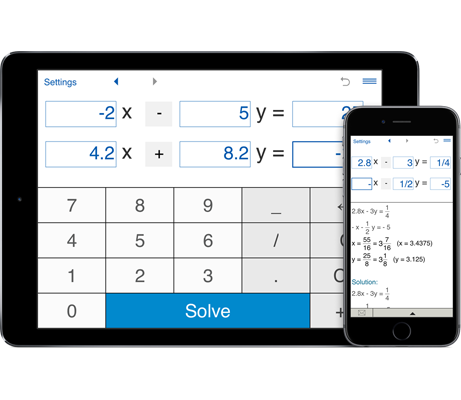# Solving systems of two linear equations in two variables

With this calculator you can easily solve systems of two linear equations in two variables step by step.

## Solving systems of two linear equations in two variables

#### Calculator to solve systems of linear equations in 2 variables with steps

The system of two linear equations with two unknowns has the form:

a11x1 + a12x2 = b1
a21x1 + a22x2 = b2

where x1 and x2 are the unknowns, a11, ..., a22 are the coefficients of the system and b1 and b2 are the constant terms.

To solve a system of two equations with two unknowns using system calculator, simply enter the coefficients of the system and press 'Solve'.

There are several methods for solving a 2x2 system of linear equations.

#### Solving 2x2 systems of linear equations using Cramer's rule

Consider the application of Cramer's rule to solve the system of two equations with 2 variables.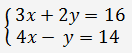As the system below has 2 rows and 2 columns and its determinant is non-zero, Cramer's rule may be applied.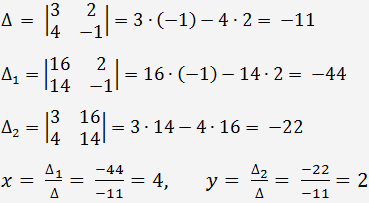#### Solving systems of 2 linear equations in 2 variables by Gaussian Elimination

In the Gaussian Elimination method you eliminate variables by transforming the system of equations into row-echelon form by means of row operations. Then the system is solved by back-substitution.

Let's solve the following system of equations using Gaussian eliminationDivide the first equation by 3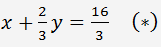Multiply (*) by 4 and add -1 times to the second equation. We get the following system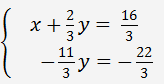From the second equation y=2. Substitution this into the first equation gives x.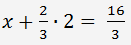x=4, y=2.

#### Solving a system of two linear equations in two variables with substitution method

The main idea of the substitution method is to solve one of the variables in terms of the others (it does not matter which equation we choose) and then substitute the result into another equation.

Consider the following system:Solving the second equation for y gives y = 4x - 14, and substituting this into the first equation gives x = (16-2(4x-14))/3.

Solving the first equation we get x = 4.

Substituting x = 4 into the second equation gives y = 2.